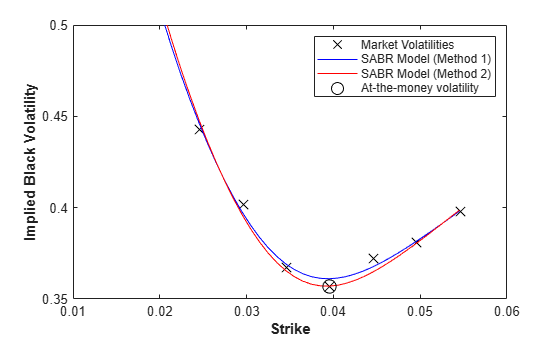Calibrate SABR Model Using Analytic Pricer

This example shows how to use two different methods to calibrate a SABR stochastic volatility model from market implied Black volatilities. Both approaches use the SABR analytic pricer.

Load Market Implied Black Volatility Data

This example sets up hypothetical market implied Black volatilities for European swaptions over a range of strikes before calibration. The swaptions expire in three years from the Settle date and have five-year swaps as the underlying instrument. The rates are expressed in decimals. (Changing the units affects the numerical value and interpretation of the Alpha parameter in the SABR model.)

Load the market implied Black volatility data for swaptions expiring in three years.

Settle = datetime(2013, 7, 10);
ZeroDates = Settle + [calmonths([1 2 3 6 9]) calyears([1 2 3 4 5 6 7 8 9 10 12])]';
ZeroRates = [0.25 0.3 0.33 0.42 0.8 0.9 1.1 1.2 ...
1.8 2.2 2.4 2.71 2.95 3.02 3.24 3.58]'/100;
Compounding = 1;
ZeroCurve = ratecurve("zero",Settle,ZeroDates,ZeroRates,'Compounding',Compounding);
ExerciseDate = datetime(2016, 7, 10);
MarketStrikes = [2.46 2.96 3.46 3.96 4.46 4.96 5.46]'/100;
MarketVolatilities = [44.3 40.2 36.7 35.7 37.2 38.1 39.8]'/100;

At the time of Settle, define the underlying forward rate and the at-the-money volatility.

CurrentForwardValue = MarketStrikes(4)
CurrentForwardValue = 0.0396
ATMVolatility = MarketVolatilities(4)
ATMVolatility = 0.3570

Method 1: Calibrate Alpha, Rho, and Nu Directly

You can calibrate the Alpha, Rho, and Nu model parameters directly. Set the value of Beta either by fitting historical market volatility data or by choosing a value appropriate for the market . For this example, use the value 0.5.

% Define the predetermined Beta.
Beta1 = 0.5;

After fixing the value of $\beta$ (Beta), fit the parameters $\alpha$ (Alpha), $\rho$ (Rho), and $\nu$ (Nu) directly. The Optimization Toolbox™ function lsqnonlin generates the parameter values that minimize the squared error between the market volatilities and the volatilities computed by the SABR analytic pricer.

% Calibrate Alpha, Rho, and Nu.
objFun = @(X) MarketVolatilities - ...
volatilities(finpricer("Analytic", 'Model', ...
finmodel("SABR", 'Alpha', X(1), 'Beta', Beta1, 'Rho', X(2), 'Nu', X(3)), ...
'DiscountCurve', ZeroCurve), ExerciseDate, CurrentForwardValue, MarketStrikes);

X = lsqnonlin(objFun, [0.5 0 0.5], [0 -1 0], [Inf 1 Inf]);
Local minimum found.

Optimization completed because the size of the gradient is less than
the value of the optimality tolerance.
Alpha1 = X(1);
Rho1 = X(2);
Nu1 = X(3);

Method 2: Calibrating Rho and Nu by Implying Alpha from At-The-Money Volatility

You can also use an alternative calibration method. Set the value of $\beta$ (Beta) as in the first method.

Beta2 = 0.5;

Next you fit the parameters $\rho$ (Rho) and $\nu$ (Nu) directly while $\alpha$ (Alpha) is implied from the market at-the-money volatility. Models calibrated using this method produce at-the-money volatilities that are equal to market quotes. This approach is widely used in swaptions, where at-the-money volatilities are quoted most frequently and are important to match. To imply $\alpha$ (Alpha) from market at-the-money volatility (${\sigma }_{ATM}$), the following cubic polynomial is solved for $\alpha$ (Alpha), and the smallest positive real root is selected .

$\frac{\left(1-\beta {\right)}^{2}T}{24{F}^{\left(2-2\beta \right)}}{\alpha }^{3}+\frac{\rho \beta \nu T}{4{F}^{\left(1-\beta \right)}}{\alpha }^{2}+\left(1+\frac{2-3{\rho }^{2}}{24}{\nu }^{2}T\right)\alpha -{\sigma }_{ATM}{F}^{\left(1-\beta \right)}=0$

Here:

$F$ is the current forward value

$T$ is the year fraction to maturity.

Fit the parameters by defining an anonymous function.

% Year fraction from Settle to option maturity.
T = yearfrac(Settle, ExerciseDate, 1);

% This function solves the SABR at-the-money volatility equation as a
% polynomial of Alpha.
alpharoots = @(Rho,Nu) roots([...
(1 - Beta2)^2*T/24/CurrentForwardValue^(2 - 2*Beta2) ...
Rho*Beta2*Nu*T/4/CurrentForwardValue^(1 - Beta2) ...
(1 + (2 - 3*Rho^2)*Nu^2*T/24) ...
-ATMVolatility*CurrentForwardValue^(1 - Beta2)]);

% This function converts at-the-money volatility into Alpha by picking the
% smallest positive real root.
atmVol2SabrAlpha = @(Rho,Nu) min(real(arrayfun(@(x) ...
x*(x>0) + realmax*(x<0 || abs(imag(x))>1e-6), alpharoots(Rho,Nu))));

The function atmVol2SabrAlpha converts at-the-money volatility into $\alpha$ (Alpha) for a given set of $\rho$ (Rho) and $\nu$ (Nu). This function is then used in the objective function to fit the parameters $\rho$ (Rho) and $\nu$ (Nu).

% Calibrate Rho and Nu (while converting at-the-money volatility into Alpha
% using atmVol2SabrAlpha).

objFun = @(X) MarketVolatilities - ...
volatilities(finpricer("Analytic", 'Model', finmodel("SABR", ...
'Alpha', atmVol2SabrAlpha(X(1), X(2)), 'Beta', Beta2, 'Rho', X(1), 'Nu', X(2)), ...
'DiscountCurve', ZeroCurve), ExerciseDate, CurrentForwardValue, MarketStrikes);

X = lsqnonlin(objFun, [0 0.5], [-1 0], [1 Inf]);
Local minimum found.

Optimization completed because the size of the gradient is less than
the value of the optimality tolerance.
Rho2 = X(1);
Nu2 = X(2);

The calibrated parameter $\alpha$ (Alpha) is computed using the calibrated parameters $\rho$ (Rho) and $\nu$ (Nu).

% Obtain final Alpha from at-the-money volatility using calibrated
% parameters.
Alpha2 = atmVol2SabrAlpha(Rho2, Nu2);

% Display calibrated parameters.
C = {Alpha1 Beta1 Rho1 Nu1;Alpha2 Beta2 Rho2 Nu2};
CalibratedPrameters = cell2table(C,...
'VariableNames',{'Alpha' 'Beta' 'Rho' 'Nu'},...
'RowNames',{'Method 1';'Method 2'})
CalibratedPrameters=2×4 table
Alpha      Beta      Rho        Nu
________    ____    _______    _______

Method 1    0.060203    0.5     0.19131    0.85327
Method 2    0.058851    0.5     0.18901    0.88627

Use Calibrated Models

Use the calibrated models to compute new volatilities at any strike value.

Compute volatilities for models calibrated using Method 1 and Method 2 and plot the results.

PlottingStrikes = (1.75:0.1:5.50)'/100;

% Compute volatilities for model calibrated by Method 1.
SABR_Model_Method_1 = finmodel("SABR", ...
'Alpha', Alpha1, 'Beta', Beta1, 'Rho', Rho1, 'Nu', Nu1);

ComputedVols1 = volatilities(finpricer("Analytic", ...
'Model', SABR_Model_Method_1, 'DiscountCurve', ZeroCurve), ...
ExerciseDate, CurrentForwardValue, PlottingStrikes);

% Compute volatilities for model calibrated by Method 2.
SABR_Model_Method_2 = finmodel("SABR", ...
'Alpha', Alpha2, 'Beta', Beta2, 'Rho', Rho2, 'Nu', Nu2);

ComputedVols2 = volatilities(finpricer("Analytic", ...
'Model', SABR_Model_Method_2, 'DiscountCurve', ZeroCurve), ...
ExerciseDate, CurrentForwardValue, PlottingStrikes);

figure;
plot(MarketStrikes,MarketVolatilities,'xk',...
PlottingStrikes,ComputedVols1,'b', ...
PlottingStrikes,ComputedVols2,'r', ...
CurrentForwardValue,ATMVolatility,'ok',...
'MarkerSize',10);
xlim([0.01 0.06]);
ylim([0.35 0.5]);
xlabel('Strike', 'FontWeight', 'bold');
ylabel('Implied Black Volatility', 'FontWeight', 'bold');
legend('Market Volatilities', 'SABR Model (Method 1)',...
'SABR Model (Method 2)', 'At-the-money volatility');The model calibrated using Method 2 reproduces the market at-the-money volatility (marked with a circle) exactly.

References

 Hagan, Patrick S., Deep Kumar, Andrew S. Lesniewski, and Diana E. Woodward. "Managing Smile Risk." Wilmott Magazine. (January 2002): 84–108.

 West, Graeme. "Calibration of the SABR Model in Illiquid Markets." Applied Mathematical Finance. 12, no. 4 (December 2005): 371–385.